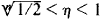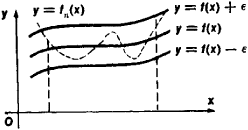# Uniform Convergence

(redirected from Uniform convergence theorem)
Also found in: Acronyms.

## uniform convergence

[′yü·nə‚fȯrm kən′vər·jəns]
(mathematics)
A sequence of functions {ƒn (x)} converges uniformly on E to ƒ(x) if given ε > 0 there is an N such that |ƒn (x) - ƒ(x)| < ε="" for="" all="">x in E provided n > N.
McGraw-Hill Dictionary of Scientific & Technical Terms, 6E, Copyright © 2003 by The McGraw-Hill Companies, Inc.
The following article is from The Great Soviet Encyclopedia (1979). It might be outdated or ideologically biased.

## Uniform Convergence

an important special case of convergence. A sequence of functions fn (x) (n = 1, 2, 3,…) is said to converge uniformly on a given set to the limit function f(x) if, for every ∊ > 0, there exists a number N = N(∊) such that, when η > N, ǀ f(x) – fn (x)ǀ < ∊ for all points x in the set.

For example, the sequence of functions fn(x) = xn converges uniformly on the closed interval [0, 1 /2] to the limit f(x) = 0. To show that this is true, let us take n > In (1/∊)/In 2. It then follows that ǀf(x)–fn (x)ǀ ≤ (1/2)n < ∊ for all x, 0 ≤ x ≤ 1/2. This sequence of functions, however, does not converge uniformly on the interval [0, 1]. Here, the limit function is f(x) = 0 for 0 ≤ x< 1 and f(1) = 1. The reason for the failure to converge uniformly is that for arbitarily large η there exist points η that satisfy the inequalitiesand for which ǀf (η) - fn(η)ǀ = ηn > 1/2.

The notion of uniform convergence admits of a simple geometric interpretation. The uniform convergence of a sequence of functions fn(x) on some closed interval to the function f(x) means that, for any ∊ > 0, all curves y = fn (x) with large enough n will be located within a strip that is 2∊ in width and is bounded by the curves y = f(x) ± ∊ for any x in the interval (see Figure 1).Figure 1

Uniformly converging sequences of functions have a number of important properties. For example, the limit of a uniformly converging sequence of continuous functions is also continuous. On the other hand, the example given above shows that the limit of a sequence of functions that does not converge uniformly may be discontinuous. An important role is played in mathematical analysis by Weierstrass’ theorem, which states that every function continuous on a closed interval can be represented as the limit of a uniformly converging sequence of polynomials. (SeeAPPROXIMATION AND INTERPOLATION OF FUNCTIONS.)